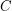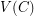Importance: High ✭✭✭
 Author(s): Thomassen, Carsten
 Subject: Graph Theory » Basic Graph Theory » » Cycles
 Keywords: chord connectivity cycle
 Posted by: mdevos on: November 12th, 2007
Conjecture   Ifis a 3-connected graph, every longest cycle inhas a chord.

A chord of a cycleis an edgeso that, but both ends ofare in. Longest cycles are of great interest in basic graph theory, and this appealing conjecture suggests a very simple property they should share - at least in 3-connected graphs.

In dense graphs, this conjecture is easy to verify - for instance, ifis Hamiltonian, it is trivially true. More interestingly, Thomassen [T] proved that his conjecture is true for cubic graphs using a clever sufficient condition for Hamiltonicity (based on Thomason's lollipop method) combined with a pretty theorem of Fleischner and Steibitz (cycle plus triangles graphs are 3-colorable).

Other work on this conjecture has focused on graphs embedded in surfaces. Zhang [Z] has proved the conjecture for planar graphs of minimum degree four, Li and Zhang have proved the conjecture for graphs in the projective plane of minimum degree four [LZ1] and for 4-connected graphs in the klein bottle or torus [LZ2]. Finally, Kawarabayashi, Niu, and Zhang [KNZ] have shown the conjecture for 4-connected graphs on a fixed surface with sufficiently high face-width.

## Bibliography

[KNZ] K. Kawarabayashi, J. Niu, C. Q. Zhang, Chords of longest circuits in locally planar graphs. European J. Combin. 28 (2007), no. 1, 315--321. MathSciNet

[LZ1] X. Li, C. Q. Zhang, Chords of longest circuits in 3-connected graphs. Discrete Math. 268 (2003), no. 1-3, 199--206. MathSciNet

[LZ2] X. Li, C. Q. Zhang, Chords of longest circuits of graphs embedded in torus and Klein bottle. J. Graph Theory 43 (2003), no. 1, 1--23. MathSciNet.

[T2] C. Thomassen, Chords of longest cycles in cubic graphs. J. Combin. Theory Ser. B 71 (1997), no. 2, 211--214. MathSciNet.

[Z] C. Q. Zhang, Longest cycles and their chords. J. Graph Theory 11 (1987), no. 4, 521--529. MathSciNet.

* indicates original appearance(s) of problem.